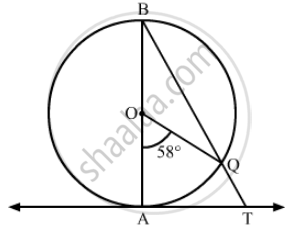Advertisement Remove all ads

# In the Given Figure, Ab is a Diameter of a Circle with Centre O and at is a Tangent. If ∠ Aoq = 58º, Find ∠ Atq. - Mathematics

Short Note

In the given figure, AB is a diameter of a circle with centre O and AT is a tangent. If $\angle$ AOQ = 58º, find  $\angle$ ATQ.Advertisement Remove all ads

#### Solution

It is given that $\angle$ AOQ = 58º.

We know that the angle subtended by an arc at the centre is twice the angle subtended by it any point on the remaining part of the circle.

∴$\angle ABQ = \frac{1}{2}\angle AOQ = \frac{1}{2} \times 58^o = 29^o$

Now, AT is the tangent and OA is the radius of the circle through the point of contact A.

$\therefore \angle OAT = 90^o$    (Tangent at any point of a circle is perpendicular to the radius through the point of contact)

In ∆ABT,

$\angle BAT + \angle ABT + \angle ATB = 180^o$   (Angle sum property)
$\Rightarrow 90^o + 29^o + \angle ATB = 180^o \left( \angle BAT = \angle OAT and \angle ABT = \angle ABQ \right)$
$\Rightarrow \angle ATB = 180^o - 119^o = 61^o$
$\therefore \angle ATQ = \angle ATB = 61^o$
Is there an error in this question or solution?
Advertisement Remove all ads

#### APPEARS IN

RD Sharma Class 10 Maths
Chapter 8 Circles
Exercise 8.2 | Q 43 | Page 39
Advertisement Remove all ads
Advertisement Remove all ads
Share
Notifications

View all notifications

Forgot password?